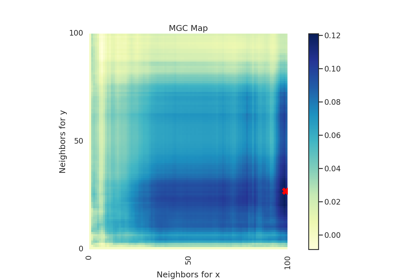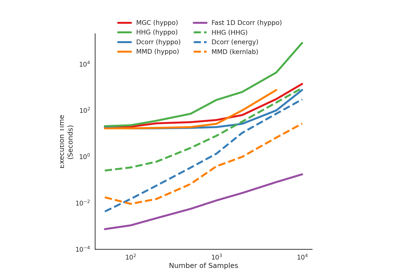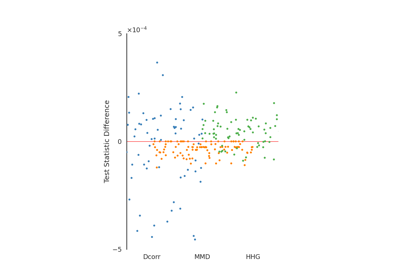# Dcorr¶

class hyppo.independence.Dcorr(compute_distance='euclidean', bias=False, **kwargs)

Distance Correlation (Dcorr) test statistic and p-value.

Dcorr is a measure of dependence between two paired random matrices of not necessarily equal dimensions. The coefficient is 0 if and only if the matrices are independent. It is an example of an energy distance.

Parameters
• compute_distance (str, callable, or None, default: "euclidean") -- A function that computes the distance among the samples within each data matrix. Valid strings for compute_distance are, as defined in sklearn.metrics.pairwise_distances,

• From scikit-learn: ["euclidean", "cityblock", "cosine", "l1", "l2", "manhattan"] See the documentation for scipy.spatial.distance for details on these metrics.

• From scipy.spatial.distance: ["braycurtis", "canberra", "chebyshev", "correlation", "dice", "hamming", "jaccard", "kulsinski", "mahalanobis", "minkowski", "rogerstanimoto", "russellrao", "seuclidean", "sokalmichener", "sokalsneath", "sqeuclidean", "yule"] See the documentation for scipy.spatial.distance for details on these metrics.

Set to None or "precomputed" if x and y are already distance matrices. To call a custom function, either create the distance matrix before-hand or create a function of the form metric(x, **kwargs) where x is the data matrix for which pairwise distances are calculated and **kwargs are extra arguements to send to your custom function.

• bias (bool, default: False) -- Whether or not to use the biased or unbiased test statistics.

• **kwargs -- Arbitrary keyword arguments for compute_distance.

Notes

The statistic can be derived as follows:

Let $$x$$ and $$y$$ be $$(n, p)$$ samples of random variables $$X$$ and $$Y$$. Let $$D^x$$ be the $$n \times n$$ distance matrix of $$x$$ and $$D^y$$ be the $$n \times n$$ be the distance matrix of $$y$$. The distance covariance is,

$\mathrm{Dcov}^b_n (x, y) = \frac{1}{n^2} \mathrm{tr} (D^x H D^y H)$

where $$\mathrm{tr} (\cdot)$$ is the trace operator and $$H$$ is defined as $$H = I - (1/n) J$$ where $$I$$ is the identity matrix and $$J$$ is a matrix of ones. The normalized version of this covariance is distance correlation 1 and is

$\mathrm{Dcorr}^b_n (x, y) = \frac{\mathrm{Dcov}^b_n (x, y)} {\sqrt{\mathrm{Dcov}^b_n (x, x) \mathrm{Dcov}^b_n (y, y)}}$

This is a biased test statistic. An unbiased alternative also exists, and is defined using the following: Consider the centering process where $$\mathbb{1}(\cdot)$$ is the indicator function:

$C^x_{ij} = \left[ D^x_{ij} - \frac{1}{n-2} \sum_{t=1}^n D^x_{it} - \frac{1}{n-2} \sum_{s=1}^n D^x_{sj} + \frac{1}{(n-1) (n-2)} \sum_{s,t=1}^n D^x_{st} \right] \mathbb{1}_{i \neq j}$

and similarly for $$C^y$$. Then, this unbiased Dcorr is,

$\mathrm{Dcov}_n (x, y) = \frac{1}{n (n-3)} \mathrm{tr} (C^x C^y)$

The normalized version of this covariance 2 is

$\mathrm{Dcorr}_n (x, y) = \frac{\mathrm{Dcov}_n (x, y)} {\sqrt{\mathrm{Dcov}_n (x, x) \mathrm{Dcov}_n (y, y)}}$

The p-value returned is calculated using a permutation test using hyppo.tools.perm_test. The fast version of the test uses hyppo.tools.chi2_approx.

When the data is 1 dimension and the distance metric is Euclidean, and even faster version of the algorithm is run (computational complexity is $$\mathcal{O}(n \log n)$$) 3.

References

1

Gábor J. Székely, Maria L. Rizzo, and Nail K. Bakirov. Measuring and testing dependence by correlation of distances. The Annals of Statistics, 35(6):2769–2794, December 2007. doi:10.1214/009053607000000505.

2

Gábor J. Székely and Maria L. Rizzo. Partial distance correlation with methods for dissimilarities. The Annals of Statistics, 42(6):2382–2412, December 2014. doi:10.1214/14-AOS1255.

3

Arin Chaudhuri and Wenhao Hu. A fast algorithm for computing distance correlation. Computational Statistics & Data Analysis, 135:15–24, July 2019. doi:10.1016/j.csda.2019.01.016.

Methods Summary

 Helper function that calculates the Dcorr test statistic. Dcorr.test(x, y[, reps, workers, auto, ...]) Calculates the Dcorr test statistic and p-value.

Dcorr.statistic(x, y)

Helper function that calculates the Dcorr test statistic.

Parameters

x,y (ndarray) -- Input data matrices. x and y must have the same number of samples. That is, the shapes must be (n, p) and (n, q) where n is the number of samples and p and q are the number of dimensions. Alternatively, x and y can be distance matrices, where the shapes must both be (n, n).

Returns

stat (float) -- The computed Dcorr statistic.

Dcorr.test(x, y, reps=1000, workers=1, auto=True, perm_blocks=None, random_state=None)

Calculates the Dcorr test statistic and p-value.

Parameters
• x,y (ndarray) -- Input data matrices. x and y must have the same number of samples. That is, the shapes must be (n, p) and (n, q) where n is the number of samples and p and q are the number of dimensions. Alternatively, x and y can be distance matrices, where the shapes must both be (n, n).

• reps (int, default: 1000) -- The number of replications used to estimate the null distribution when using the permutation test used to calculate the p-value.

• workers (int, default: 1) -- The number of cores to parallelize the p-value computation over. Supply -1 to use all cores available to the Process.

• auto (bool, default: True) -- Automatically uses fast approximation when n and size of array is greater than 20. If True, and sample size is greater than 20, then hyppo.tools.chi2_approx will be run. Parameters reps and workers are irrelevant in this case. Otherwise, hyppo.tools.perm_test will be run. If x and y have p equal to 1 and compute_distance set to 'euclidean', then and $$\mathcal{O}(n \log n)$$ version is run.

• perm_blocks (None or ndarray, default: None) -- Defines blocks of exchangeable samples during the permutation test. If None, all samples can be permuted with one another. Requires n rows. At each column, samples with matching column value are recursively partitioned into blocks of samples. Within each final block, samples are exchangeable. Blocks of samples from the same partition are also exchangeable between one another. If a column value is negative, that block is fixed and cannot be exchanged.

Returns

Examples

>>> import numpy as np
>>> from hyppo.independence import Dcorr
>>> x = np.arange(25)
>>> y = x
>>> stat, pvalue = Dcorr().test(x, y)
>>> '%.1f, %.2f' % (stat, pvalue)
'1.0, 0.00'


In addition, the inputs can be distance matrices. Using this is the, same as before, except the compute_distance parameter must be set to None.

>>> import numpy as np
>>> from hyppo.independence import Dcorr
>>> x = np.ones((10, 10)) - np.identity(10)
>>> y = 2 * x
>>> dcorr = Dcorr(compute_distance=None)
>>> stat, pvalue = dcorr.test(x, y)
>>> '%.1f, %.2f' % (stat, pvalue)
'0.0, 1.00'


## Examples using hyppo.independence.Dcorr¶